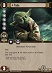Math Is Fun Forum

Discussion about math, puzzles, games and fun.   Useful symbols: ÷ × ½ √ ∞ ≠ ≤ ≥ ≈ ⇒ ± ∈ Δ θ ∴ ∑ ∫  π  -¹ ² ³ °

You are not logged in.

#1 2016-03-13 02:58:19

MemberRegistered: 2013-01-22
Posts: 149

Fast way to find primes.

Take your number, p, which you want to find out if it's prime or not.
Square root it. Multiply all the primes below the square root together.
Minus p from this number and see if there are any common factors between the two. If there is p is not prime, if there isn't p is prime.

Example:

p=51
square root p=7ish
2*3*5*7=210
210-51=159 common factor=3
51 is not prime

p=53
square root p=7ish
2*3*5*7=210
210-53=157 no common factors
53 is prime.

Note: It is impossible if the two numbers add up to a no. factorable by everything, for one to be factorable by a number and the other not. They must either both be factorable or both not.

"Time not important. Only life important." - The Fifth Element 1997

Offline

#2 2016-03-13 04:53:58

MemberRegistered: 2013-01-22
Posts: 149

Re: Fast way to find primes.

I'm not sure but I'm guessing this would be a faster way to compute finding primes. I.e. using Euclid's algorithm.
See............

https://en.wikipedia.org/wiki/Euclidean_algorithm

"Time not important. Only life important." - The Fifth Element 1997

Offline

#3 2016-03-13 20:41:47

mathaholic
MemberFrom: Earth
Registered: 2012-11-29
Posts: 3,251

Re: Fast way to find primes.

I see the technique. I tried it in several numbers and it worked.

Mathaholic | 10th most active poster | Maker of the 350,000th post | Person | rrr's classmateOffline

#4 2016-03-13 23:53:11

MemberRegistered: 2013-01-22
Posts: 149

Re: Fast way to find primes.

A= all the primes below

multiplied together
If (A-p) and p do not share a common factor then p = prime.

"Time not important. Only life important." - The Fifth Element 1997

Offline

#5 2016-03-14 01:13:45

bobbym
bumpkinFrom: Bumpkinland
Registered: 2009-04-12
Posts: 109,606

Re: Fast way to find primes.

This is called the wheel.

In mathematics, you don't understand things. You just get used to them.
If it ain't broke, fix it until it is.
Always satisfy the Prime Directive of getting the right answer above all else.

Offline

#6 2016-10-22 09:49:22

Lehona
Member
Registered: 2016-10-22
Posts: 16

Re: Fast way to find primes.

I'm not sure but I'm guessing this would be a faster way to compute finding primes. I.e. using Euclid's algorithm.
See............

https://en.wikipedia.org/wiki/Euclidean_algorithm

Primality Tests are usually done on numbers in the range of 2^1024 to 2^2048 (or much, much bigger numbers, when it's simply about "finding a prime", not crypto). Enumerating (and multiplying!) all the primes below those numbers is an insurmountable task.

If enumerating all primes was easy, RSA would be useless :-p

Edit: I just saw this thread is kinda old (still first page), I hope this doesn't count as necromancyLast edited by Lehona (2016-10-22 09:50:03)

Offline

#7 2019-11-20 02:17:05

RatnaPrabhu
Member
Registered: 2019-11-19
Posts: 6

Re: Fast way to find primes.

CHIKA OFILI Test for Divisibility by 7

For Centuries All Top Mathematicians would have had a Go at finding a Test for Divisibility by 7.

Now, a 12 year old Chika Olifi has come out with a very Simple Test.

Consider your Number as AB, where B is the last Digit and A is the rest of the Digits considered as a Single Number.
Chika Ofili Test is to Calculate A + 5B. If that is Divisible by 7, then our Original Number is Divisible by 7.

For Example, . . . Consider 588 . . . A is 58 and B is 8 . . .  A + 5B is 58 + 5 * 8 = 98 . . . this being Divisible by 7, it means 588 is Divisible by 7.

This can be Proved by a few simple steps, which our Maths Fans can try out.

Offline

#8 2019-11-20 14:38:54

Monox D. I-Fly
MemberFrom: Indonesia
Registered: 2015-12-02
Posts: 2,000

Re: Fast way to find primes.

RatnaPrabhu wrote:

CHIKA OFILI Test for Divisibility by 7

For Centuries All Top Mathematicians would have had a Go at finding a Test for Divisibility by 7.

Now, a 12 year old Chika Olifi has come out with a very Simple Test.

Consider your Number as AB, where B is the last Digit and A is the rest of the Digits considered as a Single Number.
Chika Ofili Test is to Calculate A + 5B. If that is Divisible by 7, then our Original Number is Divisible by 7.

For Example, . . . Consider 588 . . . A is 58 and B is 8 . . .  A + 5B is 58 + 5 * 8 = 98 . . . this being Divisible by 7, it means 588 is Divisible by 7.

This can be Proved by a few simple steps, which our Maths Fans can try out.

That would be useful to answer some circular questions.

Actually I never watch Star Wars and not interested in it anyway, but I choose a Yoda card as my avatar in honor of our great friend bobbym who has passed away.
May his adventurous soul rest in peace at heaven.

Offline

#9 2020-10-16 15:32:01

Knewlogik
Member
Registered: 2020-05-11
Posts: 24

Re: Fast way to find primes.

Did you know that the number one or number three the number 9 and the number 27 if you continue to add 30 too it as many times as you want and I'm pretty sure can never be a prime 21 +30=51,3+30=33,27+30=57,9+30=39if you noticed none of those are Prime's and any those four numbers you could continue to as 30 2 it's answer forever and now don't never be a prime meaning every third number ending in 1/3 7 or 9 and that form could never be a prime it's eliminate a lot of them

Offline